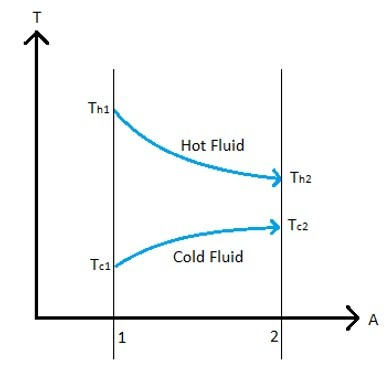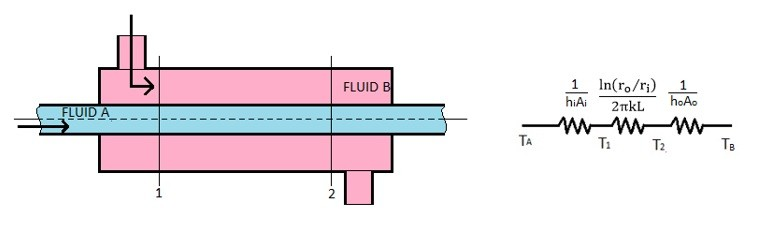# Effectiveness – NTU Method

As heat is transferred from one fluid to another fluid in a heat exchanger one fluid will rise in temperature while the other fluid falls in temperature. In the introduction article on heat exchangers the heat transfer between the two fluids was expressed as the following equation.

(Eq 1)  $q=UAΔT_{overall}$

U = Overall heat transfer coefficient

A = surface area for heat transfer consistent with the definition of U

This equation only considers the maximum temperature of the warm fluid and the minimum temperature of the cold fluid. It does not consider how the two fluid temperatures will change as they pass through the heat exchanger. Below is a temperature graph that shows the temperature profile of the two fluids for a simple two pipe heat exchanger if the fluid flow is in parallel.On the other hand the temperature graph below shows the temperature profile of the two fluids for simple two pipe heat exchanger when the flow of the two fluids is a counter flow.The image below is an example of a simple two pipe heat exchanger.From the two temperature graphs you can see how the temperature of the fluids will change as it passes through the heat exchanger. Depending on how large that change is will effect the accuracy of equation 1. One method that can be used to take this into consideration is the Log Mean Temperature Difference (LMTD). However, this method requires you to know what the input and output temperature of the fluid is. Another method that can be used is the effectiveness NTU method. To use the effectiveness NTU method you will first need to find the capacity rate of both fluids. This can be found by using the equation below.

(Eq 2)  $C_{fluid}=\dot{m}c$

m = mass flow rate

c = specific heat of the fluid

The capacity rate ratio of the heat exchanger can be now be found using the following equation.

(Eq 3)  $C=\frac{C_{min}}{C_{max}}$

Cmin is the capacity rate of the fluid with lowest capacity rate while Cmax is the capacity rate of the fluid with the highest capacity rate.

Now that the capacity rate of the fluids in the heat exchanger have been determined the NTU value can be found. The NTU value is known as the number of transfer units and is represented by the equation below.

(Eq 4) $N=NTU = \frac{UA}{C_{min}}$

Finally, the effectiveness of the heat transfer needs to be determined. The effectiveness of a heat transfer is the ratio of the actual heat transfer that will occur over the maximum heat transfer that could occur as seen in the equation below.

(Eq 5)  $ε=\frac{actual~heat~transfer}{max~heat~transfer}$

To find the maximum heat transfer of the heat exchanger equation 1 could be used or the equation below could be used.

(Eq 6)  $q_{max}=C_{min}(T_{h~insert}-T_{c~inlet})$

To find the actual heat transfer of the heat exchanger the equation below would be used.

(Eq 7)  $q=εq_{max}$

The actual effectiveness of the heat exchanger is dependent on the fluid flow in the heat exchanger and the type of heat exchanger. Below is a list of equations that apply to different situations. To use the equations you will need to first determine the NTU value, N, of the heat exchanger and you will need to find capacity rate ratio, C, of the heat exchanger.

## Double Pipe Parallel Flow

(Eq 8)  $ε=\frac{1-e^{N(1+C)}}{1+C}$

## Double Pipe Counter Flow

(Eq 9)  $ε=\frac{1-e^{-N(1-C)}}{1-Ce^{-N(1-C)}}$

## Cross Flow Both Fluids Unmixed

(Eq 10) $ε=1-exp\left[ \frac{e^{NCn}-1}{Cn} \right]$

Where n = N-0.22

## Cross Flow Both Fluids Mixed

(Eq 11)  $ε = \left[ \frac{1}{1-e^-N} + \frac{C}{1-e^{NC}} – \frac{1}{N} \right]^{-1}$

## Cross Flow CMax Mixed, CMin Unmixed

(Eq 12) $ε = \frac{1}{C}\{1-exp[-C(1-e^{-N}) ] \}$

## Cross Flow CMax Unmixed, CMin Mixed

(Eq 13)  $ε=1-exp\left\{-\frac{1}{C} [1-e^{-NC}] \right \}$

## Shell and Tube: One Shell Pass, 2, 4, 6 Tube Passes

(Eq 14) $ε = 2 \left\{ 1+C+(1+C^2)^{1/2} \left(\frac{1+e^{-N(1+C^2)^{1/2}}} {1-e^{-N(1+C^2)^{1/2}}} \right) \right\}^{-1}$